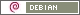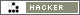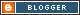Contact me sending an e-mail (antispam defense activated)Use byte multiplicative suffix size

# Use byte multiplicative suffix size

Sandro Tosi, 28 January 2007

When you need to handle byte sizes as user input, could be easier, from a user point of view, to insert the value 650M instead of the exact value in bytes (681574400, for you information).

The Java method below does exactly this work: it tries to convert the input parameter in a number of bytes, even if it's using multiplicative suffix.

Here is the code (highlighted with java2html), a wrapping (executable) class around it is available here:

 ``` /** * Tries to convert size, expressed even with multiplicative * suffix, into a number of bytes. * * For example, 120M will be come 125829120 (i.e. 120 x 1024 x * 1024), and so on. If the parameter is a simple number * (i.e. 512), no conversion is performed. * * Supported suffices are: 'k', 'K' (for KiloBytes), 'm', 'M' (for * MegaBytes), 'g', 'G' (for GigaBytes). * * In case of any error during number conversion, a * NumberFormatException is raised. * * @param sizeS string representing the size * @return byte value of size passed as parameter */ public long byteSuffixSize(String sizeS) throws NumberFormatException { long size = 0; String quant = ""; char unit; try { // Try to convert the string to a long, since parameter // could be a simple number size = (new Integer(sizeS)).longValue(); } catch (NumberFormatException nfe) { // it's a controlled situation; it could still be a // multiplicative suffix try { // Take every char except the last quant = sizeS.substring(0, sizeS.length()-1); // Take the last char, maybe the multiplicative suffix unit = sizeS.charAt(sizeS.length()-1); size = (new Integer(quant)).longValue(); // Based on the suffix, evaluate the correct size switch (unit){ case 'K': case 'k': size = size * 1024; break; case 'M': case 'm': size = size * 1024 * 1024; break; case 'G': case 'g': size = size * 1024 * 1024 * 1024; break; default: // If it's not in previous cases, than it's not a // multiplicative suffix, so die throw new NumberFormatException(); } } catch (Exception e) { // In case of any error, die raising a // NumberFormatException throw new NumberFormatException(e.toString()); } // inner try..catch } // outer try..catch return size; } // byteSuffixSize ```

Drop me an email, if you'd like to provide some comments.